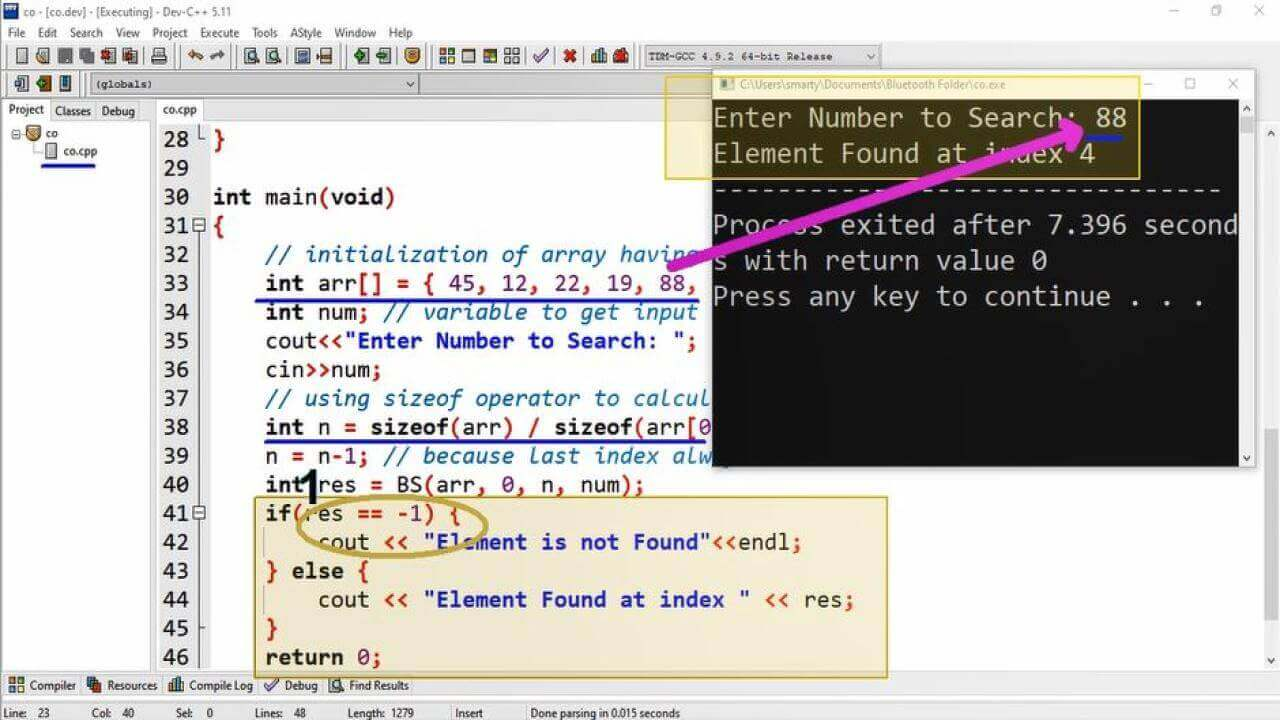# CPP Binary Search Using Recursion Program Example

## Source Code:

#include <iostream>
using namespace std;

// function name BS means binary search
int BS(int arr[], int left, int right, int num)
{
//base condition for recursion
if (right >= left) {
/* calculating mid of array to
divide it into two parts */

int mid = left + (right - left) / 2;

// If the element found at the mid
if (arr[mid] == num)
return mid; // returning mid index

/* if element is smaller then we have to
find it in left subarray */

if (arr[mid] > num)
return BS(arr, left, mid - left, num);

/* if element is smaller then we have to
find it in left subarray */

return BS(arr, mid + left, right, num);
}
return -1;
}

int main(void)
{
// initialization of array having 10 elements
int arr[] = { 45, 12, 22, 19, 88, 54, 66, 12, 40, 77 };
int num; // variable to get input of number to search
cout<<"Enter Number to Search: ";
cin>>num;
// using sizeof operator to calculate elements of array
int n = sizeof(arr) / sizeof(arr);
n = n-1; // because last index always = total elements - 1
int res = BS(arr, 0, n, num);
if(res == -1) {
} else {
cout << "Element Found at index " << res;
}
return 0;
}

## Output:## Working:

Binary search algorithm is implemented using recursion, because this algorithm involves continous divion of provided data set into two halves. Word binary means two. So that the algorithm get the mid of the input array and check whether the mid element is equal to the provided search key, if not then algorithm find it in the subsequent parts of the array.

This algorithm involve the following procedure recursively.

1. Sort Array (Learn about Sorting Techniques)
2. Divide array into two halves
3. Match search key with value at mid index
4. If value match with mid index value then return index
5. Else if search key is greater then mid value the skip the lower half
6. Else if search key is smaller then mid value the skip the upper half
7. Repeat from step 2

Here base condition for this recursive algorithm is right > left. This means that array can further be divided into two halves. An when this condition will false then array cannot further divided so that recursion will terminate. In case of value match this algorithm will return its index. Otherwise unsuccessfull completion algorithm will return -1.

In main function we have initialized an array of 10 distinct elements. A variable of int type is declared to input serach key from user. After declaration process input is taken from the user using cout and cin objects. BS (binary Search) algorithm is called providing array and search key.

Finally we check if the return value of BS algorithm if it is -1 then we are displaying message "Element is not Found" other wise we will print the message including the index at which we found value.